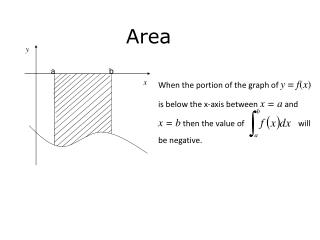DownloadDownload PresentationArea

# Area

Download Presentation## Area

- - - - - - - - - - - - - - - - - - - - - - - - - - - E N D - - - - - - - - - - - - - - - - - - - - - - - - - - -
##### Presentation Transcript

1. y a b x When the portion of the graph of y = f(x) is below the x-axis between x = a and x = b then the value of will be negative. Area

2. Area Entrapped between two curves

3. Example Find the finite area enclosed between the graph of and

4. Example • Find the points of intersection for the graphs of and • Hence find the finite area enclosed between the graph of and

5. y 0 x Example • The graph drawn above is of the line and the curve • Find the points of intersection for the graphs and • Hence find the finite area enclosed between the graph of and • for x > 0.